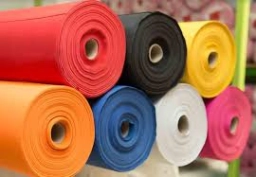# A meter

A meter of cloth costs Rs 16 1/2. Find the cost of 8 3/4 m of fabric.

x =  144 38 = 1155/8 Rs

### Step-by-step explanation:Did you find an error or inaccuracy? Feel free to write us. Thank you!

Tips for related online calculators
Need help calculating sum, simplifying, or multiplying fractions? Try our fraction calculator.
Need help with mixed numbers? Try our mixed-number calculator.
Do you want to convert length units?